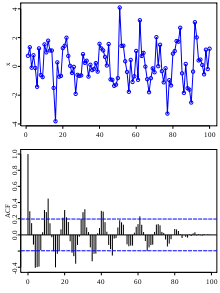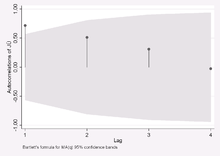# Correlogram

Not to be confused with Scatterplot.A plot showing 100 random numbers with a "hidden" sine function, and an autocorrelation (correlogram) of the series on the bottom.Example for a correlogram

In the analysis of data, a correlogram is an image of correlation statistics. For example, in time series analysis, a correlogram, also known as an autocorrelation plot, is a plot of the sample autocorrelationsversus(the time lags).

If cross-correlation is used, the result is called a cross-correlogram. The correlogram is a commonly used tool for checking randomness in a data set. This randomness is ascertained by computing autocorrelations for data values at varying time lags. If random, such autocorrelations should be near zero for any and all time-lag separations. If non-random, then one or more of the autocorrelations will be significantly non-zero.

In addition, correlograms are used in the model identification stage for Box–Jenkins autoregressive moving average time series models. Autocorrelations should be near-zero for randomness; if the analyst does not check for randomness, then the validity of many of the statistical conclusions becomes suspect. The correlogram is an excellent way of checking for such randomness.

Sometimes, corrgrams, color-mapped matrices of correlation strengths in multivariate analysis, are also called correlograms.

## Applications

The correlogram can help provide answers to the following questions:

• Are the data random?
• Is an observation related to an adjacent observation?
• Is an observation related to an observation twice-removed? (etc.)
• Is the observed time series white noise?
• Is the observed time series sinusoidal?
• Is the observed time series autoregressive?
• What is an appropriate model for the observed time series?
• Is the modelvalid and sufficient?

• Is the formulavalid?

## Importance

Randomness (along with fixed model, fixed variation, and fixed distribution) is one of the four assumptions that typically underlie all measurement processes. The randomness assumption is critically important for the following three reasons:

• Most standard statistical tests depend on randomness. The validity of the test conclusions is directly linked to the validity of the randomness assumption.
• Many commonly used statistical formulae depend on the randomness assumption, the most common formula being the formula for determining the standard deviation of the sample mean:where s is the standard deviation of the data. Although heavily used, the results from using this formula are of no value unless the randomness assumption holds.

• For univariate data, the default model isIf the data are not random, this model is incorrect and invalid, and the estimates for the parameters (such as the constant) become nonsensical and invalid.

## Estimation of autocorrelations

The autocorrelation coefficient at lag h is given bywhere ch is the autocovariance functionand c0 is the variance functionThe resulting value of rh will range between -1 and +1.

### Alternate estimate

Some sources may use the following formula for the autocovariance function:Although this definition has less bias, the (1/N) formulation has some desirable statistical properties and is the form most commonly used in the statistics literature. See pages 20 and 49-50 in Chatfield for details.

## Statistical inference with correlograms

In the same graph one can draw upper and lower bounds for autocorrelation with significance level:withas the estimated autocorrelation at lag.

If the autocorrelation is higher (lower) than this upper (lower) bound, the null hypothesis that there is no autocorrelation at and beyond a given lag is rejected at a significance level of. This test is an approximate one and assumes that the time-series is Gaussian.

In the above, z1-α/2 is the quantile of the normal distribution; SE is the standard error, which can be computed by Bartlett's formula for MA(l) processes:forIn the picture above we can reject the null hypothesis that there is no autocorrelation between time-points which are adjacent (lag=1). For the other periods one cannot reject the null hypothesis of no autocorrelation.

Note that there are two distinct formulas for generating the confidence bands:

1. If the correlogram is being used to test for randomness (i.e., there is no time dependence in the data), the following formula is recommended:where N is the sample size, z is the quantile function of the standard normal distribution and α is the significance level. In this case, the confidence bands have fixed width that depends on the sample size.

2. Correlograms are also used in the model identification stage for fitting ARIMA models. In this case, a moving average model is assumed for the data and the following confidence bands should be generated:where k is the lag. In this case, the confidence bands increase as the lag increases.

## Software

Correlograms are available in most general purpose statistical software programs. In R, the function acf and pacf can be used to produce such a plot.

## References

1. Friendly, Michael (19 August 2002). "Corrgrams: Exploratory displays for correlation matrices" (PDF). The American Statistician. Taylor & Francis. 56 (4): 316–324. doi:10.1198/000313002533. Retrieved 19 January 2014.
2. "CRAN - Package corrgram". cran.r-project.org. 29 August 2013. Retrieved 19 January 2014.
3. "Quick-R: Correlograms". statmethods.net. Retrieved 19 January 2014.This article incorporates public domain material from the National Institute of Standards and Technology website http://www.nist.gov.